DraftKings NFC Championship Odds
+330
3.3 to 1Buccaneers
20.0% implied probability

+450
4.5 to 1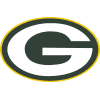Packers
15.7% implied probability

+450
4.5 to 1Rams
15.7% implied probability

+750
7.5 to 149ers
10.1% implied probability

+900
9 to 1Cowboys
8.6% implied probability

+1100
11 to 1Eagles
7.2% implied probability

+2000
20 to 1Cardinals
4.1% implied probability

+2000
20 to 1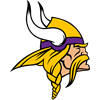Vikings
4.1% implied probability

+2500
25 to 1Saints
3.3% implied probability

+2500
25 to 1Commanders
3.3% implied probability

+6000
60 to 1Panthers
1.4% implied probability

+6000
60 to 1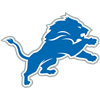Lions
1.4% implied probability

+6000
60 to 1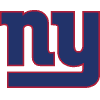Giants
1.4% implied probability

+6000
60 to 1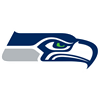Seahawks
1.4% implied probability

+7000
70 to 1Bears
1.2% implied probability

+8000
80 to 1Falcons
1.1% implied probability